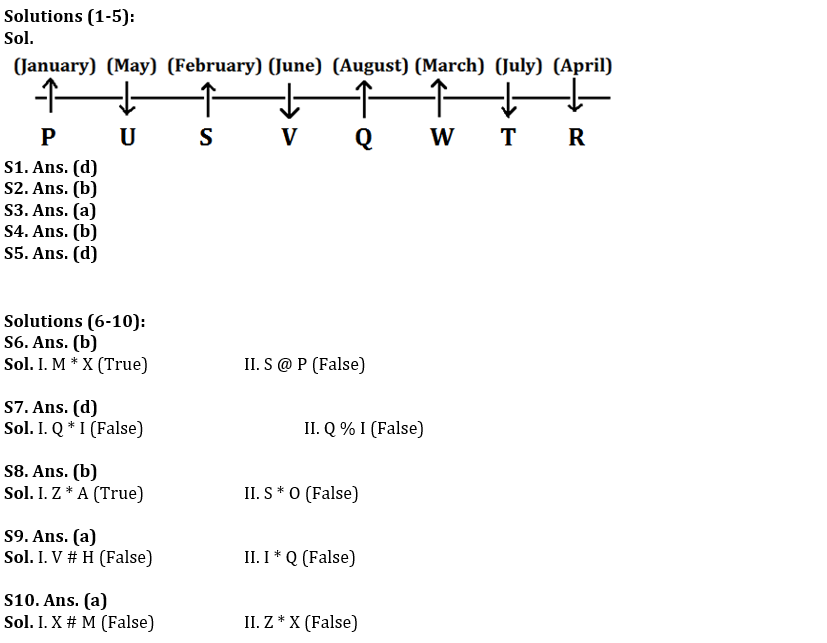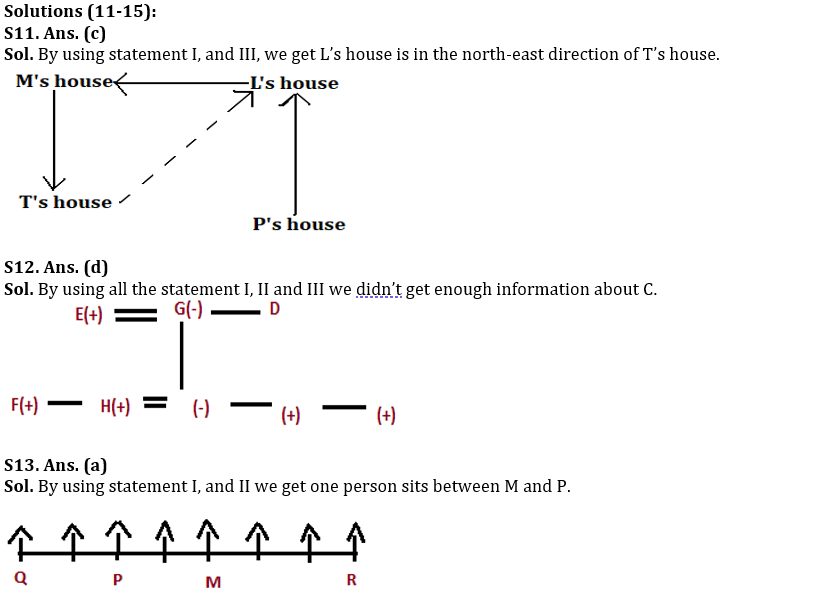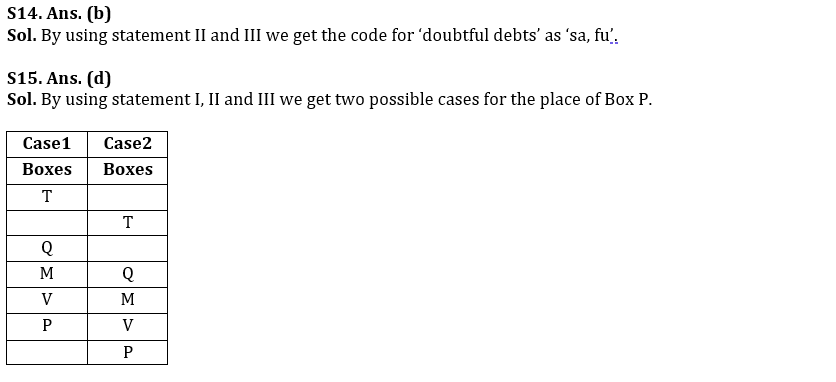Latest Banking jobs   »   Reasoning Quiz For RBI Grade B...

# Reasoning Quiz For RBI Grade B Phase 1 2023-5th March

Directions (1-5): Read the given information carefully and answer the given questions.
Eight friends sit in a straight line. Some of them face north while some of them face south direction. All of them were born in different months starting from January to August of the same year and on same date.
R who sits at the extreme end of the row and faces south. W is not an immediate neighbour of the oldest person and faces opposite to U and R. Only three persons sit between R and V who was born in June. T is not an immediate neighbour of V and sits second to the right of Q. S sits second to the right of P who is the oldest person in the group. W is the third oldest person in the group. P is not an immediate neighbour of R and V. U was born in May and sits fourth to the left of the one who was born in March. T faces same direction as U but opposite to Q and S. R is four months older than Q. Second oldest person sits immediate right of the one who is third youngest.

Q1. Who among the following second youngest person in the group?
(a) S
(b) W
(c) U
(d) T
(e) None of these

Q2. Who among the following sits third to the right of S?
(a) Q
(b) W
(c) U
(d) P
(e) None of these

Q3. S was born in which of the following month?
(a) February
(b) January
(c) March
(d) August
(e) None of these

Q4. Which of the following statement is not true about V?
(a) V sits third to the right of the one who was born in January
(b) V was born in March
(c) W sits second to the left of V
(d) V is facing south
(e) None is true

Q5. How Many persons sit between R and U?
(a) One
(b) Two
(c) Three
(d) More than four
(e) None

Directions (6-10): In the following questions, the symbols %, &, #, * and @ are used with the following meaning as illustrated below-
‘P # Q’ means ‘P is neither greater than nor equal to Q’
‘P * Q’ means ‘P is neither equal to nor smaller than Q’
‘P % Q’ means ‘P is neither smaller than nor greater than Q’
‘P @ Q’ means ‘P is not smaller than Q’
‘P & Q’ means ‘P is not greater than Q’
Now in each of the following questions assuming the given statement to be true, find which of the conclusions given below them is/are definitely true and give your answer accordingly.

Q6. Statements: M * O @ W # S; O * E % P @ X
Conclusions: I. M * X
II. S @ P
(a) None is true
(b) Only I is true
(c) Only II is true
(d) Either I or II is true
(e) Both are true

Q7. Statements: I & K & N % Q * W @ C * P % V
Conclusions: I. Q * I
II. Q % I
(a) None is true
(b) Both are true
(c) Only II is true
(d) Either I or II is true
(e) Only I is true

Q8. Statements: O @ A # R % S & M; L * M % C & Z
Conclusions: I. Z * A
II. S * O
(a) None is true
(b) Only I is true
(c) Only II is true
(d) Either I or II is true
(e) Both are true

Q9. Statements: V & Q % S % L * H * P # I
Conclusions: I. V # H
II. I * Q
(a) None is true
(b) Only I is true
(c) Only II is true
(d) Either I or II is true
(e) Both are true

Q10. Statements: D @ M & Z * K # P; D * L * N % X
Conclusions: I. X # M
II. Z * X
(a) None is true
(b) Only I is true
(c) Only II is true
(d) Either I or II is true
(e) Both are true

Directions (11-15): Each of the questions below consists of a question and three statements numbered I, II and III given below it. You have to decide whether the data provided in the statements are sufficient to answer the question. Read both the statements and give answer.
(a) If the data in statement I and II are sufficient to answer the question.
(b) If the data in statement II and III are sufficient to answer the question.
(c) If the data in statement I and III are sufficient to answer the question.
(d) If the data in all the statements I, II and III together are not sufficient to answer the question.
(e) If all the statements I, II and III are necessary to answer the questions.

Q11. What is the direction of L’s house with respect to T’s house?
(I) M’s house is in the west of L’s house which is in the north of P’s house.
(II) K’s house is in the east of T’s house which is in the west of U’s house.
(III) T’s house is in the south of M’s house.

Q12. Eight persons A, B, C, D, E, F, G and H are members of a family of three generations. How is C related to the mother-in-law of H?
(I) F is the only brother of H who is the son-in-law of G.
(II) E is married to G who is sister of D.
(III) E has only three children and one daughter.

Q13. Eight persons are sitting in a straight row facing north. How many persons sit between M and P if M sits to the right of P?
(I) only four persons sit between P and R who sits at the end of the row. M sits to the left of R.
(II) Three persons sit between M and Q who does not sit near to R and P.
(III) M does not sit at any ends of the row.

Q14. What is the code for ‘doubtful debts’ in the given code language?
(I) If ‘provision for bad debts’ is coded as ‘lo sa mr ru’
(II) ‘provision for doubtful debts’ is coded as ‘ru sa lo fu’
(III) ‘doubtful bad debts are NPA’ is coded as ‘sa mr fu gd’

Q15. Seven boxes are kept above one another. What is the position of Box P from the top?
(I) Only three boxes are kept between box T and box V which is kept one of the places above box T.
(II) Only one box is kept between box V and box Q. Box P is kept one of the places below box Q but not immediately below.
(III) Only one box is kept between box P and Box M.

Solutions## FAQs

### What is the selection process of the Bank Clerk?

There are 4 sections in the RBI Grade B Phase 1 Exam i.e. English Language, General Awareness, Quantitative Aptitude & Reasoning.

#### Congratulations!Union Budget 2023-24: Free PDF